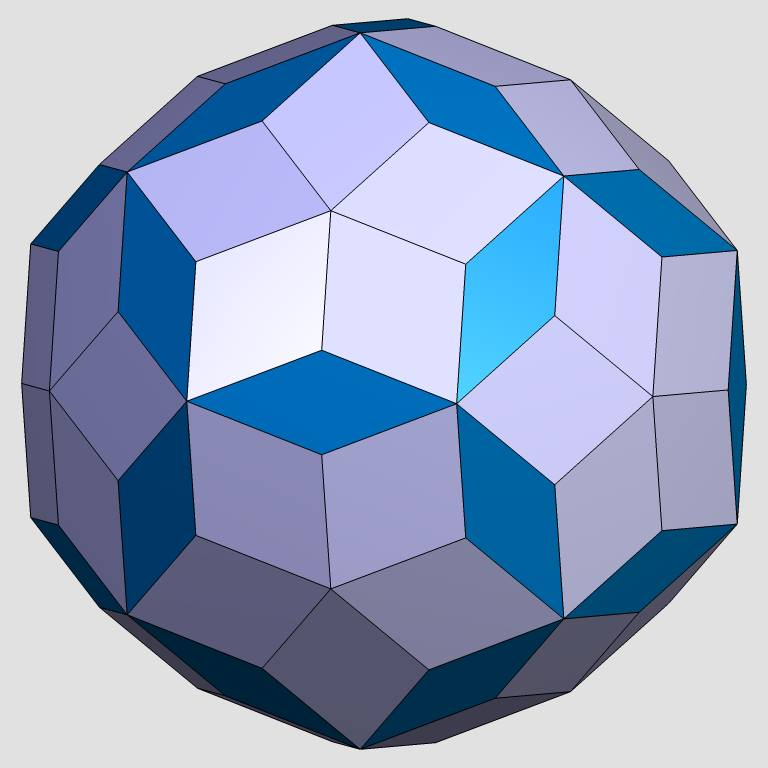Acronym ... Name rhombic enneacontahedron©© Vertex figure [r5], [(r,r')3], [R2,R'] General of army (is itself convex) Colonel of regiment (is itself locally convex) ExternallinksThe fatter rhombs {(r,R)2} have vertex angles r = arccos(1/3) = 70.528779° resp. R = arccos(-1/3) = 109.471221°. Esp. rr : RR = sqrt(2) = 1.414214.
The thinner rhombs {(r',R')2} have vertex angles r' = arccos[sqrt(5)/3] = 41.810315° resp. R' = arccos[-sqrt(5)/3] = 138.189685°. Esp. r'r' : R'R' = (3+sqrt(5))/2 = 2.618034.
Moreover the second pic above neatly displays the geometry of the 2 types of rhombs in relation to the ike, there being used as vertex representant.

All a, b, c, and d edges, provided in the below description, only qualify as pseudo edges wrt. the full polyhedron.

Incidence matrix according to Dynkin symbol

abo3oco5ood&#zx   → height = 0,
a = sqrt(20/3) = 2.581989,
b = R'R' = (sqrt(5)-1)/sqrt(3) = 0.713644,
c = RR = 2/sqrt(3) = 1.154701,
d = r'r' = (1+sqrt(5))/sqrt(3) = 1.868345

o..3o..5o..     | 12  *  * |  5   0 |  5  0  [r5]
.o.3.o.5.o.     |  * 60  * |  1   2 |  2  1  [R2,R']
..o3..o5..o     |  *  * 20 |  0   6 |  3  3  [(r,r')3]
----------------+----------+--------+------
oo.3oo.5oo.&#x  |  1  1  0 | 60   * |  2  0
.oo3.oo5.oo&#x  |  0  1  1 |  * 120 |  1  1
----------------+----------+--------+------
... oco ...&#xt |  1  2  1 |  2   2 | 60  *  {(r,R)2}
.bo ... .od&#zx |  0  2  2 |  0   4 |  * 30  {(r',R')2}